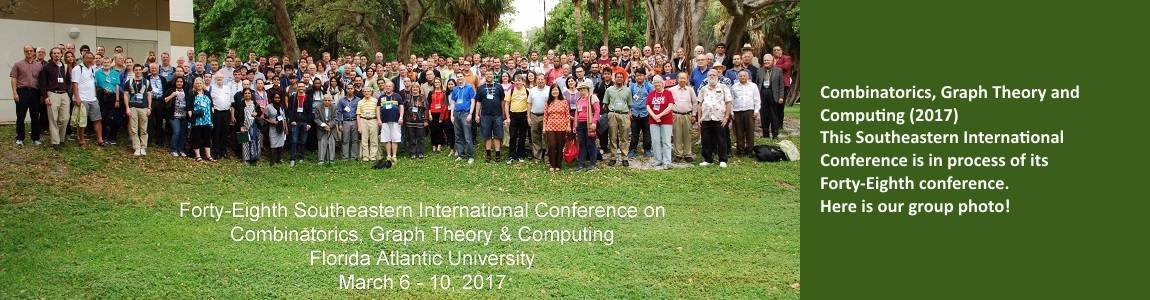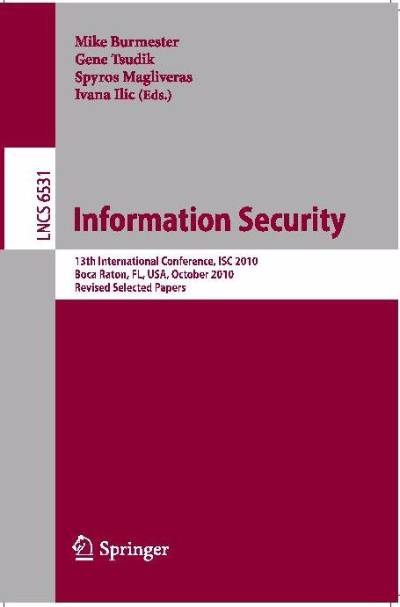# Dr. Spyros Magliveras• Professor
• Department of Mathematical Sciences
• spyros@fau.edu
• Boca Raton - SE43, Room 276

## Education

• Ph.D. in Mathematics (Group Theory),  University of Birmingham, England, 1970

## Research Interests

• Cryptology
• Finite Groups
• Combinatorics - Design Theory
• Computational Algebra
• Coding Theory
• Image Compression
• Design & Complexity of Algorithms
• Algebraic Computer Science
• Finite Geometries

My main research interests are in cryptology, algebraic combinatorics, coding theory, and algorithms and their complexity, particularly  those  related  to computational group theory.  For over 40 years I have been interested in group theoretic cryptography.   I am also interested in data compression, specifically in applications of coding theory to lossless image compression.  I am interested in combinatorial designs and related finite geometries, including t-designs, Large Sets of t-designs, Room Rectangles, mutually orthogonal Latin squares, projective planes,  orthogonal and perpendicular arrays.  I am also interested in algorithmic methods in design theory, especially those methods which lead to proofs of existence/non-existence of designs for  large t, and small lambda.  These methods include the use of groups, large knap-sack solvers, and isomorphism testing.

## Research Description

Some of the highlights include:  The invention of group theoretic Cryptosystem PGM, and cryptosystems MSTi, i=1,2,3.  The discovery of the world's first simple 6-design, and the construction of infinite families of 5-designs.  New ideas in the non-abelian discrete logarithm problem, leading to a collaboration and cryptanalysis of the foundational Tillich-Zemor hash function, the discovery of new large sets of t-designs and collaborations in the discovery of large sets of geometric t-designs, and some constructions and decompositions of well known strongly regular graphs. Research in lattice basis reduction using permutation codes and optimization techniques.

## Scholarly Activities